# Programming Tutorial:Implementing another Matlab-based Filter

This tutorial, written by Robert Oostenveld, shows you how to implement a filter based on a beamforming algorithm where the beamformer is updated in real-time, based on the covariance matrix of its input data.

## What is Matlab?

“MATLAB® is a high-level language and interactive environment that enables you to perform computationally intensive tasks faster than with traditional programming languages such as C, C++, and Fortran.”

### Advantages of Matlab

• interactive
• simple syntax
• no explicit declaration of variables and functions required
• garbage collection
• standard for neuroscience data analysis
• many toolboxes available
• many algorithms implemented
• many data visualisation tools

### Disadvantages of Matlab

• slower than compiled code
• default double precision
• not open source
• expensive
• language suitable for RAD, not so much for large projects

## Real time processing for BCI

• EEG alpha oscillation @10Hz
duration ~100 ms
decision every xx ms?
• Processing as fast as possible
"real time"
In computer science, real-time computing is the study of hardware and software systems which are subject to a "real-time constraint”, i.e., operational deadlines from event to system response.
By contrast, a non-real-time system is one for which there is no deadline, even if fast response or high performance is desired or even preferred.
• Deadline requirements vary, e.g.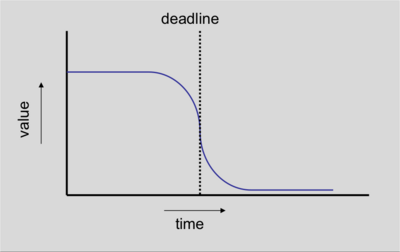continuous classification -> hard deadline
incremental evidence -> soft deadline

## Motivation for combining BCI2000 and Matlab

• rapid application development
• try out various algorithms
• offline analysis of data
• port offline analysis to online
• Matlab is fast enough for quite some computations

## Matlab in the BCI2000 pipeline

• BCI2000 filters are pipelined
• each filter is a C++ object, there exists a Matlab filter that translates the C++ interface into Matlab
constructor -- define states and parameters, start Matlab engine
Preflight() -- check validity of parameters
Initialize() -- get parameter values
StartRun() -- setup computational space
StopRun() -- cleanup computational space
while data is streaming
Process() -- perform actual computation
destructor -- stop Matlab engine
• correspondingly, there is a Matlab function corresponding to each C++ member function:
bci_Construct() -- define states and parameters, start Matlab engine
bci_Preflight() -- check validity of parameters
bci_Initialize() -- get parameter values
bci_StartRun() -- setup computational space
while data is streaming
bci_Process() -- process a single data block
bci_StopRun() -- cleanup computational space
destructor() -- stop Matlab engine

## Filter example: linear classification using a beamformer

Beamforming is a technique to extract source signals from a multi-channel recording. In this hands-on tutorial, we will use an adaptive spatial beamformer to compute a biophysically motivated source projection which is adapted to data covariance.

### Adaptive Spatial Filtering

Region in the brain: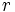$r$

Forward model: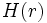$H(r)$

Assumed neural activity: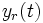$y_r(t)$

Model for the projection of the source to the channels (forward model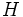$H$):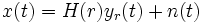$x(t)=H(r) y_r(t) + n(t)$

Estimate the strength of activity of the neural tissue at location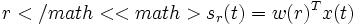$r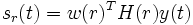$s_r(t) = w(r)^TH(r)y(t)$

An ideal spatial filter should pass activity from a location of interest with unit gain: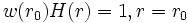$w(r_0) H(r) = 1, r=r_0$

while suppressing others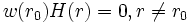$w(r_0) H(r) = 0, r\neq r_0$

However, this is not always possible, and we compute an optimal spatial filter by minimizing the variance of the filter output (source activity):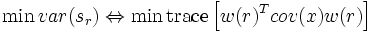$\min var(s_r) \Leftrightarrow \min \textrm{trace}\left[w(r)^T cov(x) w(r)\right]$

Two constraints: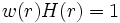$w(r) H(r) = 1$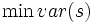$\min var(s)$

After some algebra: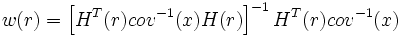$w(r)=\left[H^T(r) cov^{-1}(x) H(r)\right]^{-1} H^T(r) cov^{-1}(x)$

The adaptive filter is computed from the forward model for a given position$r$, and the covariance matrix of the data.

### Implementation with the BCI2000 Matlab filter

#### bci_StartRun function

function bci_StartRun

% shared BCI2000 Parameters and states are global variables.
global bci_Parameters bci_States

% the following variables are used in the computation, and are needed over multiple iterations
global fsample nchans nsamples
global sum_covariance sum_count
global norm_s norm_ss norm_n
global H C w

fsample  = sscanf(bci_Parameters.SamplingRate{1}, '%fHz');
nchans   = sscanf(bci_Parameters.SourceCh{1}, '%d');
nsamples = sscanf(bci_Parameters.SampleBlockSize{1}, '%d');

% these are for the accumulated normalization
norm_n  = 0;
norm_s  = 0;
norm_ss = 0;

% these are for the accumulated data covariance estimate
sum_covariance = zeros(nchans, nchans);
sum_count      = 0;

% this is the forward model, which describes how the source projects onto the channels
% in real applications the forward model would be computed using a biophysical model
% but here the source projects equally strong onto all channels
H = ones(nchans,1)/nchans;

% these are empty to start with
C = zeros(nchans, nchans);
w = zeros(1,nchans);


#### bci_Process Function

function out_signal = bci_Process( in_signal )

<...>

flt_signal = in_signal;

% apply baseline correction
for i=1:nchan
flt_signal(i,:) = flt_signal(i,:) - mean(flt_signal(i,:));
end

% compute the covariance using a running sum
sum_covariance = sum_covariance + flt_signal*flt_signal';
sum_count      = sum_count      + 1;

% compute the beamformer spatial filter
C = sum_covariance/sum_count;
w = inv(H' * inv(C) * H) * H' * inv(C);

% apply the beamformer spatial filter to the data
out_signal = w * flt_signal;

% compute the total power in the signal for the present block
out_signal = sqrt(sum(out_signal.^2));

% we could in principle stop here, but normalization of the control signal is required as well
% the normalization could also be done with standard BCI2000 filter

% if the signal is all zero, then the inverse covariance cannot be computed
% which results in a filter output that is not a number (nan)
if ~isnan(out_signal)
% compute the running sum of the beamformer power
for i=1:numel(out_signal)
norm_n  = norm_n  + 1;
norm_s  = norm_s  + out_signal(i);
norm_ss = norm_ss + out_signal(i)^2;
end
end

% compute the normalized output
norm_avg   = norm_s / norm_n;
norm_std   = sqrt(norm_ss/norm_n - norm_s^2/norm_n^2);
out_signal = (out_signal - norm_avg)/norm_std;


### Beamformer source reconstruction

• adaptive spatial filter
• implementation in few lines of Matlab code
• recursive updating of covariance
• output used as control signal

## Conclusions

• Small-batch processing in Matlab
• Incremental algorithms
• Within the pipeline
• Usefull for Rapid Application Development
• Fast for Research & Development
• Fast enough (?) for online applications
• once proven, port algorithm to C++